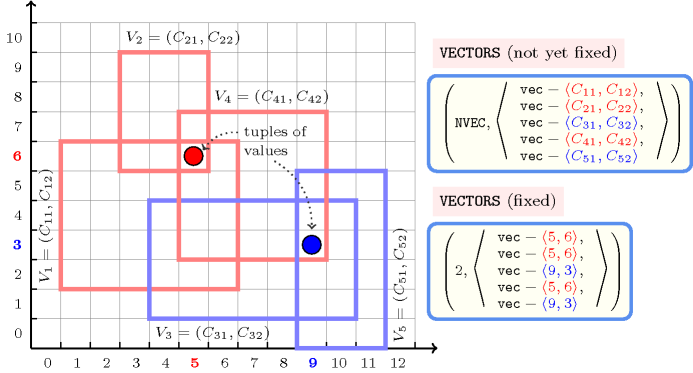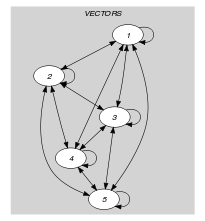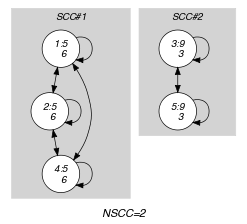## 5.290. nvector

Origin

Introduced by G. Chabert as a generalisation of $\mathrm{𝚗𝚟𝚊𝚕𝚞𝚎}$

Constraint

$\mathrm{𝚗𝚟𝚎𝚌𝚝𝚘𝚛}\left(\mathrm{𝙽𝚅𝙴𝙲},\mathrm{𝚅𝙴𝙲𝚃𝙾𝚁𝚂}\right)$

Synonyms

$\mathrm{𝚗𝚟𝚎𝚌𝚝𝚘𝚛𝚜}$, $\mathrm{𝚗𝚙𝚘𝚒𝚗𝚝}$, $\mathrm{𝚗𝚙𝚘𝚒𝚗𝚝𝚜}$.

Type
 $\mathrm{𝚅𝙴𝙲𝚃𝙾𝚁}$ $\mathrm{𝚌𝚘𝚕𝚕𝚎𝚌𝚝𝚒𝚘𝚗}\left(\mathrm{𝚟𝚊𝚛}-\mathrm{𝚍𝚟𝚊𝚛}\right)$
Arguments
 $\mathrm{𝙽𝚅𝙴𝙲}$ $\mathrm{𝚍𝚟𝚊𝚛}$ $\mathrm{𝚅𝙴𝙲𝚃𝙾𝚁𝚂}$ $\mathrm{𝚌𝚘𝚕𝚕𝚎𝚌𝚝𝚒𝚘𝚗}\left(\mathrm{𝚟𝚎𝚌}-\mathrm{𝚅𝙴𝙲𝚃𝙾𝚁}\right)$
Restrictions
 $|\mathrm{𝚅𝙴𝙲𝚃𝙾𝚁}|\ge 1$ $\mathrm{𝙽𝚅𝙴𝙲}\ge \mathrm{𝚖𝚒𝚗}\left(1,|\mathrm{𝚅𝙴𝙲𝚃𝙾𝚁𝚂}|\right)$ $\mathrm{𝙽𝚅𝙴𝙲}\le |\mathrm{𝚅𝙴𝙲𝚃𝙾𝚁𝚂}|$ $\mathrm{𝚛𝚎𝚚𝚞𝚒𝚛𝚎𝚍}$$\left(\mathrm{𝚅𝙴𝙲𝚃𝙾𝚁𝚂},\mathrm{𝚟𝚎𝚌}\right)$ $\mathrm{𝚜𝚊𝚖𝚎}_\mathrm{𝚜𝚒𝚣𝚎}$$\left(\mathrm{𝚅𝙴𝙲𝚃𝙾𝚁𝚂},\mathrm{𝚟𝚎𝚌}\right)$
Purpose

$\mathrm{𝙽𝚅𝙴𝙲}$ is the number of distinct tuples of values taken by the vectors of the collection $\mathrm{𝚅𝙴𝙲𝚃𝙾𝚁𝚂}$. Two tuples of values $〈{A}_{1},{A}_{2},\cdots ,{A}_{m}〉$ and $〈{B}_{1},{B}_{2},\cdots ,{B}_{m}〉$ are $distinct$ if and only if there exist an integer $i\in \left[1,m\right]$ such that ${A}_{i}\ne {B}_{i}$.

Example
$\left(\begin{array}{c}2,〈\begin{array}{c}\mathrm{𝚟𝚎𝚌}-〈5,6〉,\hfill \\ \mathrm{𝚟𝚎𝚌}-〈5,6〉,\hfill \\ \mathrm{𝚟𝚎𝚌}-〈9,3〉,\hfill \\ \mathrm{𝚟𝚎𝚌}-〈5,6〉,\hfill \\ \mathrm{𝚟𝚎𝚌}-〈9,3〉\hfill \end{array}〉\hfill \end{array}\right)$

The $\mathrm{𝚗𝚟𝚎𝚌𝚝𝚘𝚛}$ constraint holds since its first argument $\mathrm{𝙽𝚅𝙴𝙲}=2$ is set to the number of distinct tuples of values (i.e., tuples $〈5,6〉$ and $〈9,3〉$) occurring within the collection $\mathrm{𝚅𝙴𝙲𝚃𝙾𝚁𝚂}$. Figure 5.290.1 depicts with a thick rectangle a possible initial domain for each of the five vectors and with a grey circle each tuple of values of the corresponding solution.

##### Figure 5.290.1. Possible initial domains (${C}_{11}\in \left[1,6\right]$, ${C}_{12}\in \left[2,6\right]$, ${C}_{21}\in \left[3,5\right]$, ${C}_{22}\in \left[6,9\right]$, ${C}_{31}\in \left[4,10\right]$, ${C}_{32}\in \left[1,4\right]$, ${C}_{41}\in \left[5,9\right]$, ${C}_{42}\in \left[3,7\right]$, ${C}_{51}\in \left[9,11\right]$, ${C}_{52}\in \left[0,5\right]$) and solution corresponding to the Example slot: we have two distinct vectors ($\mathrm{𝙽𝚅𝙴𝙲}=2$)Typical
 $|\mathrm{𝚅𝙴𝙲𝚃𝙾𝚁}|>1$ $\mathrm{𝙽𝚅𝙴𝙲}>1$ $\mathrm{𝙽𝚅𝙴𝙲}<|\mathrm{𝚅𝙴𝙲𝚃𝙾𝚁𝚂}|$ $|\mathrm{𝚅𝙴𝙲𝚃𝙾𝚁𝚂}|>1$
Symmetries
• Items of $\mathrm{𝚅𝙴𝙲𝚃𝙾𝚁𝚂}$ are permutable.

• Items of $\mathrm{𝚅𝙴𝙲𝚃𝙾𝚁𝚂}.\mathrm{𝚟𝚎𝚌}$ are permutable (same permutation used).

• All occurrences of two distinct tuples of values of $\mathrm{𝚅𝙴𝙲𝚃𝙾𝚁𝚂}.\mathrm{𝚟𝚎𝚌}$ can be swapped; all occurrences of a tuple of values of $\mathrm{𝚅𝙴𝙲𝚃𝙾𝚁𝚂}.\mathrm{𝚟𝚎𝚌}$ can be renamed to any unused tuple of values.

Arg. properties
• Functional dependency: $\mathrm{𝙽𝚅𝙴𝙲}$ determined by $\mathrm{𝚅𝙴𝙲𝚃𝙾𝚁𝚂}$.

• Contractible wrt. $\mathrm{𝚅𝙴𝙲𝚃𝙾𝚁𝚂}$ when $\mathrm{𝙽𝚅𝙴𝙲}=1$ and $|\mathrm{𝚅𝙴𝙲𝚃𝙾𝚁𝚂}|>0$.

• Contractible wrt. $\mathrm{𝚅𝙴𝙲𝚃𝙾𝚁𝚂}$ when $\mathrm{𝙽𝚅𝙴𝙲}=|\mathrm{𝚅𝙴𝙲𝚃𝙾𝚁𝚂}|$.

Remark

It was shown in [ChabertLorca09], [ChabertJaulinLorca09] that, finding out whether a $\mathrm{𝚗𝚟𝚎𝚌𝚝𝚘𝚛}$ constraint has a solution or not is NP-hard (i.e., the restriction to the rectangle case and to the atmost side of the $\mathrm{𝚗𝚟𝚎𝚌𝚝𝚘𝚛}$ were considered for this purpose). This was achieved by reduction from the rectangle clique partition problem.

Reformulation

Assume the collection $\mathrm{𝚅𝙴𝙲𝚃𝙾𝚁𝚂}$ is not empty (otherwise $\mathrm{𝙽𝚅𝙴𝙲}=0$). In this context, let $n$ and $m$ respectively denote the number of vectors of the collection $\mathrm{𝚅𝙴𝙲𝚃𝙾𝚁𝚂}$ and the number of components of each vector. Furthermore, let ${\alpha }_{i}=min\left(\underline{{C}_{1i}},\underline{{C}_{2i}},\cdots ,\underline{{C}_{ni}}\right)$, ${\beta }_{i}=max\left(\overline{{C}_{1i}},\overline{{C}_{2i}},\cdots ,\overline{{C}_{ni}}\right)$, ${\gamma }_{i}={\beta }_{i}-{\alpha }_{i}+1$, $\left(i\in \left[1,m\right]\right)$. By associating to each vector

$〈{C}_{k1},{C}_{k2},\cdots ,{C}_{km}〉,\left(k\in \left[1,n\right]\right)$

a variable

${D}_{k}=\sum _{1\le i\le m}\left(\left(\prod _{i

the constraint

$\mathrm{𝚗𝚟𝚎𝚌𝚝𝚘𝚛}$$\left(\mathrm{𝙽𝚅𝙴𝙲},$

$〈\mathrm{𝚟𝚎𝚌}-〈{C}_{11},{C}_{12},\cdots ,{C}_{1m}〉,$

$\mathrm{𝚟𝚎𝚌}-〈{C}_{21},{C}_{22},\cdots ,{C}_{2m}〉,$

$\cdots \cdots \cdots \cdots \cdots \cdots \cdots \cdots$

$\mathrm{𝚟𝚎𝚌}-〈{C}_{n1},{C}_{n2},\cdots ,{C}_{nm}〉〉\right)$

can be expressed in term of the constraint

$\mathrm{𝚗𝚟𝚊𝚕𝚞𝚎}$$\left(\mathrm{𝙽𝚅𝙴𝙲},〈{D}_{1},{D}_{2},\cdots ,{D}_{n}〉\right)$.

Note that the previous reformulation does not work anymore if the variables have a continuous domain, or if an overflow occurs while propagating the equality constraint ${D}_{k}={\sum }_{1\le i\le m}\left(\left({\prod }_{i (i.e., the number of components $m$ is too big).

When using this reformulation with respect to the Example slot we first introduce ${D}_{1}=1·6-3+\left(4·5-20\right)\right)=3$, ${D}_{2}=1·6-3+\left(4·5-20\right)\right)=3$, ${D}_{3}=1·3-3+\left(4·9-20\right)\right)=16$, ${D}_{4}=1·6-3+\left(4·5-20\right)\right)=3$, ${D}_{5}=1·3-3+\left(4·9-20\right)\right)=16$ and then get the constraint $\mathrm{𝚗𝚟𝚊𝚕𝚞𝚎}$$\left(2,〈3,3,16,3,16〉\right)$.

generalisation: $\mathrm{𝚗𝚟𝚎𝚌𝚝𝚘𝚛𝚜}$ (replace an equality with the number of distinct vectors by a comparison with the number of distinct nvectors).

implies: $\mathrm{𝚊𝚝𝚕𝚎𝚊𝚜𝚝}_\mathrm{𝚗𝚟𝚎𝚌𝚝𝚘𝚛}$ ($=\mathrm{𝙽𝚅𝙴𝙲}$ replaced by $\ge \mathrm{𝙽𝚅𝙴𝙲}$), $\mathrm{𝚊𝚝𝚖𝚘𝚜𝚝}_\mathrm{𝚗𝚟𝚎𝚌𝚝𝚘𝚛}$ ($=\mathrm{𝙽𝚅𝙴𝙲}$ replaced by $\le \mathrm{𝙽𝚅𝙴𝙲}$).

specialisation: $\mathrm{𝚗𝚟𝚊𝚕𝚞𝚎}$ (vector replaced by $\mathrm{𝚟𝚊𝚛𝚒𝚊𝚋𝚕𝚎}$).

Keywords
Arc input(s)

$\mathrm{𝚅𝙴𝙲𝚃𝙾𝚁𝚂}$

Arc generator
$\mathrm{𝐶𝐿𝐼𝑄𝑈𝐸}$$↦\mathrm{𝚌𝚘𝚕𝚕𝚎𝚌𝚝𝚒𝚘𝚗}\left(\mathrm{𝚟𝚎𝚌𝚝𝚘𝚛𝚜}\mathtt{1},\mathrm{𝚟𝚎𝚌𝚝𝚘𝚛𝚜}\mathtt{2}\right)$

Arc arity
Arc constraint(s)
$\mathrm{𝚕𝚎𝚡}_\mathrm{𝚎𝚚𝚞𝚊𝚕}$$\left(\mathrm{𝚟𝚎𝚌𝚝𝚘𝚛𝚜}\mathtt{1}.\mathrm{𝚟𝚎𝚌},\mathrm{𝚟𝚎𝚌𝚝𝚘𝚛𝚜}\mathtt{2}.\mathrm{𝚟𝚎𝚌}\right)$
Graph property(ies)
$\mathrm{𝐍𝐒𝐂𝐂}$$=\mathrm{𝙽𝚅𝙴𝙲}$

Graph class
$\mathrm{𝙴𝚀𝚄𝙸𝚅𝙰𝙻𝙴𝙽𝙲𝙴}$

Graph model

Parts (A) and (B) of Figure 5.290.2 respectively show the initial and final graph associated with the Example slot. Since we use the $\mathrm{𝐍𝐒𝐂𝐂}$ graph property we show the different strongly connected components of the final graph. Each strongly connected component corresponds to a tuple of values that is assigned to some vectors of the $\mathrm{𝚅𝙴𝙲𝚃𝙾𝚁𝚂}$ collection. The 2 following tuple of values $〈5,6〉$ and $〈9,3〉$ are used by the vectors of the $\mathrm{𝚅𝙴𝙲𝚃𝙾𝚁𝚂}$ collection.

##### Figure 5.290.2. Initial and final graph of the $\mathrm{𝚗𝚟𝚎𝚌𝚝𝚘𝚛}$ constraint(a) (b)